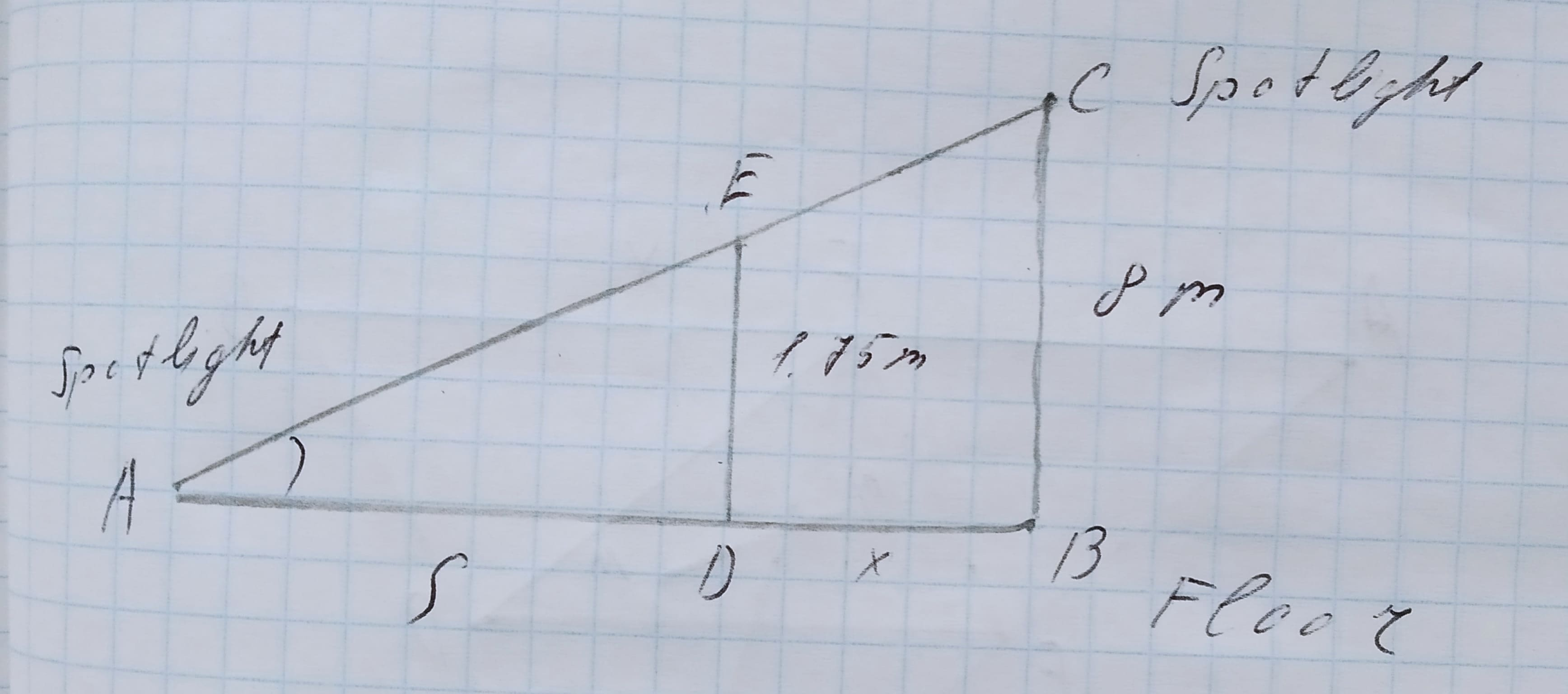# A spotlight at a building corridor is fastened to a wall 8m above the floor. A lady 1.75m tall moves away from the wall at a speed of 0.75m/s. a.)at what rate is the lenght of her shadow increasing? b.)at what speed is the tip of her shadow moving?coexpennan 2021-02-27 Answered
A spotlight at a building corridor is fastened to a wall 8m above the floor. A lady 1.75m tall moves away from the wall at a speed of $0.75\frac{m}{s}$.
a.)at what rate is the lenght of her shadow increasing?
b.)at what speed is the tip of her shadow moving?
You can still ask an expert for help

• Questions are typically answered in as fast as 30 minutes

Solve your problem for the price of one coffee

• Math expert for every subject
• Pay only if we can solve itTheodore Schwartz

Step 1
Please have a look at the picture below to understand what's going on:For the sake of clarity,
and x = distance of the lady from the wall = BD
$\frac{dx}{dt}=0.75\frac{m}{s}$
Step 2
Triangle ADE is similar to triangle ABC (AAA criterion of similarity)
Hence, $D\frac{E}{B}C=A\frac{D}{A}B$ (Corresponding sides of similar triangles are proportional)
Hence, $\frac{1.75}{8}=\frac{s}{s+x}$
Hence, $1.75\left(s+x\right)=8s$
Or, $1.75x=\left(8-1.75\right)s=6.25s$
Step 3
Part (a)
Differentiate both sides w.r.t time t to get:
$\frac{1.75dx}{dt}=\frac{6.25ds}{dt}$
Hence, the rate at which length of her shadow is increasing = $\frac{ds}{dt}=\frac{\left(\frac{1.75}{6.25}\right)dx}{dt}=\left(\frac{1.75}{6.25}\right)x0.75=0.21\frac{m}{s}$
Step 4
Part (b)
the rate at which the tip of her shadow is moving = rate t which she is moving + rate at which the length of the shadow is increasing = $\frac{dx}{dt}+\frac{ds}{dt}=0.75+0.21=0.96\frac{m}{s}$
Step 5
Part (a) $0.21\frac{m}{s}$
Part (b) $0.96\frac{m}{s}$